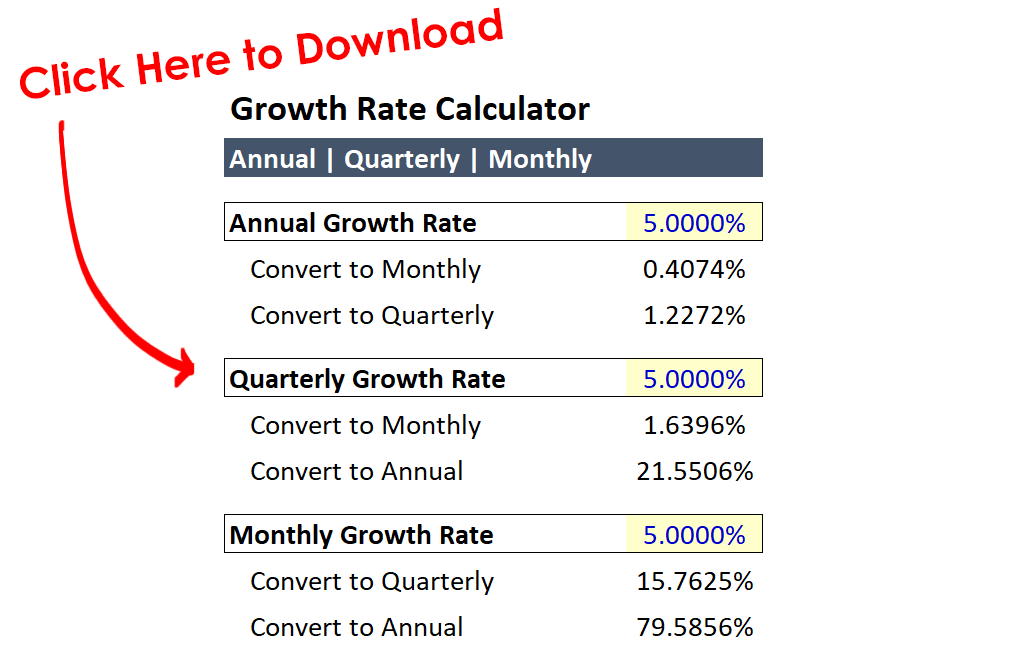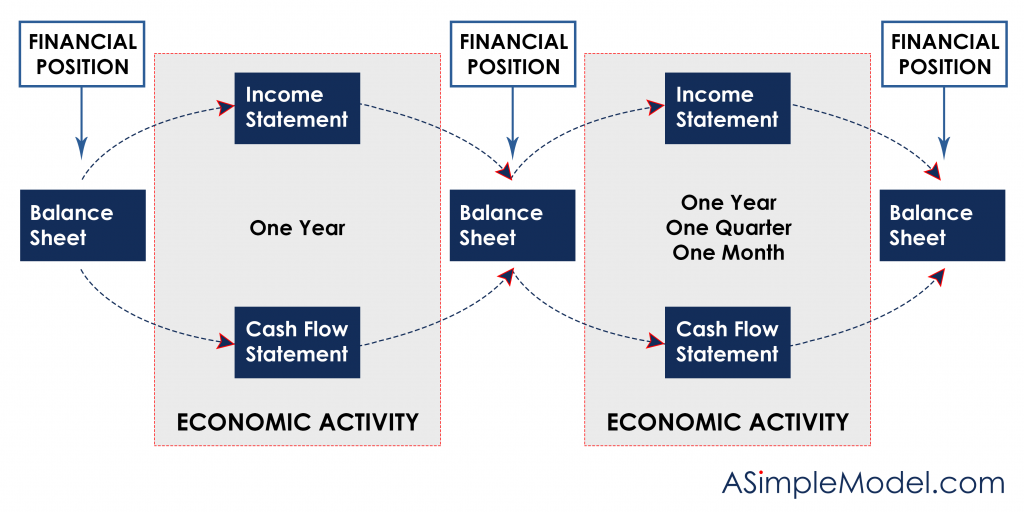Select Page

# Converting an Annual Growth Rate to a Monthly Growth Rate

• Peter Lynch

The most common error I see in financial models as it relates to growth rates is to divide an annual growth rate by 12 to arrive at the monthly growth rate. In this post we will explore the correct way to convert growth rates for all periods.

There is also an Excel template available for download that will make this calculation for you so that you can check your math (the calculator will apply the conversion to annual, quarterly and monthly periods).

The correct approach is to apply exponents, and we can explain why this is the correct approach with a simple explanation. When a value grows by 5% from one period to the next you multiply the value by 1.05. If this were to occur for 12 consecutive periods the multiplication pattern would repeat.

The simplest way to explain this is to solve for the value that when multiplied by itself 12 times returns (1 + the Annual Growth Rate). So for an annual growth rate of 5% we would take the approach that follows.And since we are solving for (1 + Growth Rate), we subtract 1 from the outcome:Formulas for Each Period Follow:

Annual To Monthly: (1 + Growth Rate)^(1/12)-1

Annual to Quarterly: (1 + Growth Rate)^(1/4)-1

Quarterly to Monthly: (1 + Growth Rate)^(1/3)-1

Quarterly to Annual: (1 + Growth Rate)^(4)-1

Monthly to Quarterly: (1 + Growth Rate)^(3)-1

Monthly to Annual: (1 + Growth Rate)^(12)-1For more information on working with monthly periods please click on this LINK (or the image below).